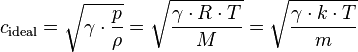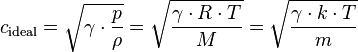# Answer Checking (Speed of Sound formula, dimensional analysis)

Phonetic

## Homework Statement

One formula for measuring the speed of sound in air is:
$v=\sqrt\frac{kTg}{m}$

v=velocity
T=temperature
g=dimensionless constant
m=average mass of an air molecule

If all units are SI, what are the units of k?

## The Attempt at a Solution

I've done a lot of Googling to first try and find formulas for the speed of sound. The most similar I've seen on Wikipedia waswith the last part of the formula being the most relevant
Cideal = ideal speed
gamma = adiabatic index
k = Boltzmann Constant
T = temperature in Kelvin
m = mass of a single molecule in kg

That makes me think that k is a placeholder for the adiabatic index or the Boltzmann Constant, but I'm not sure which (if either) it is.

## Homework Statement

In this question, the length of a wave, λ, has dimensions L, wave speed (v) has dimensons L/T, and gravitational acceleration (g) has units of L/T². Could the following be an equation for wave speed:

## Homework Equations

$v=\sqrt\frac{g}{λ}$

## The Attempt at a Solution

λ=L
v=D/T
g=L/T²

$D/T = \sqrt\frac{L/T²}{L}$

$D/T=\sqrt{T²}$

$D/T=T$

No it could not be an equation for wave speed because it is not dimensionally proportionate.

If I didn't get these right, where did I go wrong? I feel like I got the second one right, but I'm completely clueless on the first one. Thanks in advance!

Last edited:

Homework Helper

## Homework Statement

One formula for measuring the speed of sound in air is:
$v=\sqrt\frac{kTg}{m}$

v=velocity
T=temperature
g=dimensionless constant
m=average mass of an air molecule

If all units are SI, what are the units of k?

## The Attempt at a Solution

I've done a lot of Googling to first try and find formulas for the speed of sound. The most similar I've seen on Wikipedia waswith the last part of the formula being the most relevant
Cideal = ideal speed
gamma = adiabatic index
k = Boltzmann Constant
T = temperature in Kelvin
m = mass of a single molecule in kg

That makes me think that k is a placeholder for the adiabatic index or the Boltzmann Constant, but I'm not sure which (if either) it is.

No need to look for similar equations. They just wanted you to get the units of k.

you have velocity on the left side in m/s. Write down the units of the rest of units of the dimensions. Then put them into the formula and re-arrange to find k.

## Homework Statement

In this question, the length of a wave, λ, has dimensions L, wave speed (v) has dimensons L/T, and gravitational acceleration (g) has units of L/T². Could the following be an equation for wave speed:

## Homework Equations

$v=\sqrt\frac{g}{λ}$

## The Attempt at a Solution

λ=L
v=D/T
g=L/T²

$D/T = \sqrt\frac{L/T²}{L}$

$D/T=\sqrt{T²}$

$D/T=T$

No it could not be an equation for wave speed because it is not dimensionally proportionate.

If I didn't get these right, where did I go wrong? I feel like I got the second one right, but I'm completely clueless on the first one. Thanks in advance!

This one is correct.